1598887560

# Feature Engineering — deep dive into Encoding and Binning techniques

Feature engineering is the most important aspect of a data science model development. There are several categories of features in a raw dataset. Features can be text, date/time, categorical, and continuous variables. For a machine learning model, the dataset needs to be processed in the form of numerical vectors to train it using an ML algorithm.

• Feature Binning: Conversion of a continuous variable to categorical.
• Feature Encoding: Conversion of a categorical variable to numerical features.

## Feature Binning:

Binning or discretization is used for the transformation of a continuous or numerical variable into a categorical feature. Binning of continuous variable introduces non-linearity and tends to improve the performance of the model. It can be also used to identify missing values or outliers.

There are two types of binning:

• Unsupervised Binning: Equal width binning, Equal frequency binning
• Supervised Binning: Entropy-based binning

### Unsupervised Binning:

Unsupervised binning is a category of binning that transforms a numerical or continuous variable into categorical bins without considering the target class label into account. Unsupervised binning are of two categories:

### 1. Equal Width Binning:

This algorithm divides the continuous variable into several categories having bins or range of the same width.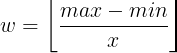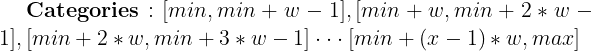``````Notations,
x = number of categories
w = width of a category
max, min = Maximum and Minimun of the list
``````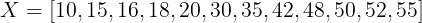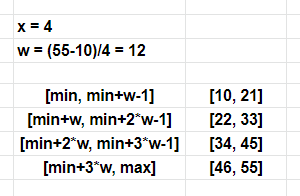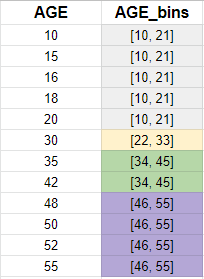#artificial-intelligence #machine-learning #feature-engineering #data-science #nlp

## Buddha Community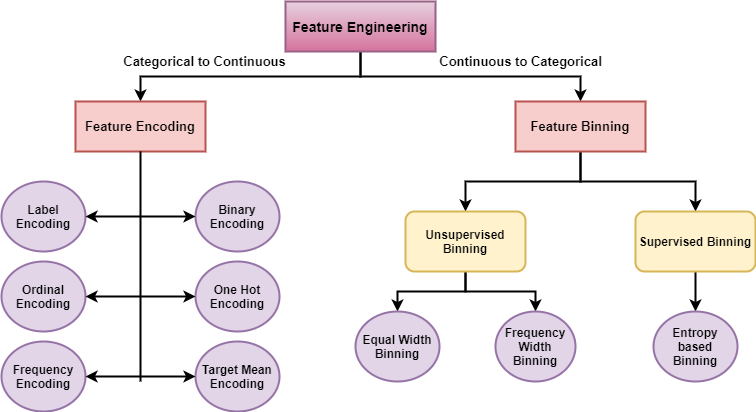1598887560

## Feature Engineering — deep dive into Encoding and Binning techniques

Feature engineering is the most important aspect of a data science model development. There are several categories of features in a raw dataset. Features can be text, date/time, categorical, and continuous variables. For a machine learning model, the dataset needs to be processed in the form of numerical vectors to train it using an ML algorithm.

• Feature Binning: Conversion of a continuous variable to categorical.
• Feature Encoding: Conversion of a categorical variable to numerical features.

## Feature Binning:

Binning or discretization is used for the transformation of a continuous or numerical variable into a categorical feature. Binning of continuous variable introduces non-linearity and tends to improve the performance of the model. It can be also used to identify missing values or outliers.

There are two types of binning:

• Unsupervised Binning: Equal width binning, Equal frequency binning
• Supervised Binning: Entropy-based binning

### Unsupervised Binning:

Unsupervised binning is a category of binning that transforms a numerical or continuous variable into categorical bins without considering the target class label into account. Unsupervised binning are of two categories:

### 1. Equal Width Binning:

This algorithm divides the continuous variable into several categories having bins or range of the same width.``````Notations,
x = number of categories
w = width of a category
max, min = Maximum and Minimun of the list
``````#artificial-intelligence #machine-learning #feature-engineering #data-science #nlp1598245080

## Feature Engineering: What is Feature Engineering?

According to a survey in Forbes, data scientists spend 80% of their time on data preparation. This shows the importance of feature engineering in data science. Here are some valuable quotes about Feature Engineering and its importance:

Coming up with features is difficult, time-consuming, requires expert knowledge. ‘Applied machine learning’ is basically feature engineering — Prof. Andrew Ng.

The features you use influence more than everything else the result. No algorithm alone, to my knowledge, can supplement the information gain given by correct feature engineering — Luca Massaron

What is Feature Engineering?

Feature engineering is the process of transforming raw data into features that better represent the underlying problem to the predictive models, resulting in an improved model accuracy on unseen data.

Basically, all machine learning algorithms use some input data to create outputs. This input data comprises features, which are usually in the form of structured columns. Algorithms require features with some specific characteristic to work properly

Having and engineering good features will allow us to most accurately represent the underlying structure of the data and therefore create the best model. Features can be engineered by decomposing or splitting features, from external data sources, or aggregating or combining features to create new features.

#data-science #feature-engineering #feature-selection #data analysis1618317562

## Top Deep Learning Development Services | Hire Deep Learning Developer

We at Inexture, strategically work on every project we are associated with. We propose a robust set of AI, ML, and DL consulting services. Our virtuoso team of data scientists and developers meticulously work on every project and add a personalized touch to it. Because we keep our clientele aware of everything being done associated with their project so there’s a sense of transparency being maintained. Leverage our services for your next AI project for end-to-end optimum services.

#deep learning development #deep learning framework #deep learning expert #deep learning ai #deep learning services1596040980

# Overview

1. Understand the requirement of feature transformation and training techniques
2. Get to know different feature transformation and scaling techniques including-
• MinMax Scaler
• Standard Scaler
• Power Transformer Scaler
• Unit Vector Scaler/Normalizer

# Introduction

In my machine learning journey, more often than not, I have found that feature preprocessing is a more effective technique in improving my evaluation metric than any other step, like choosing a model algorithm, hyperparameter tuning, etc.

Feature preprocessing is one of the most crucial steps in building a Machine learning model. Too few features and your model won’t have much to learn from. Too many features and we might be feeding unnecessary information to the model. Not only this, but the values in each of the features need to be considered as well.

We know that there are some set rules of dealing with categorical data, as in, encoding them in different ways. However, a large chunk of the process involves dealing with continuous variables. There are various methods of dealing with continuous variables. Some of them include converting them to a normal distribution or converting them to categorical variables, etc.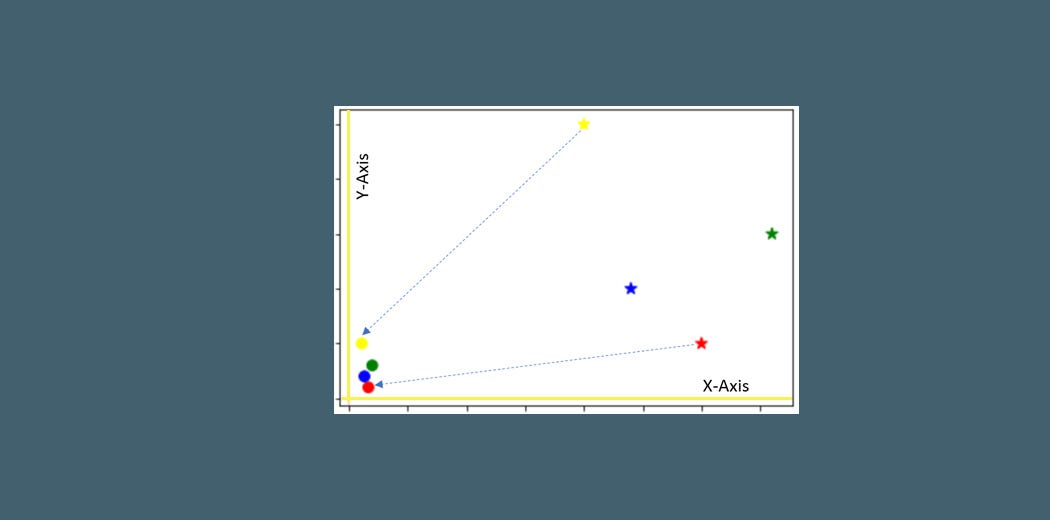There are a couple of go-to techniques I always use regardless of the model I am using, or whether it is a classification task or regression task, or even an unsupervised learning model. These techniques are:

• Feature Transformation and
• Feature Scaling.

_To get started with Data Science and Machine Learning, check out our course — _Applied Machine Learning — Beginner to Professional

1. Why do we need Feature Transformation and Scaling?
2. MinMax Scaler
3. Standard Scaler
4. MaxAbsScaler
5. Robust Scaler
6. Quantile Transformer Scaler
7. Log Transformation
8. Power Transformer Scaler
9. Unit Vector Scaler/Normalizer

## Why do we need Feature Transformation and Scaling?

Oftentimes, we have datasets in which different columns have different units — like one column can be in kilograms, while another column can be in centimeters. Furthermore, we can have columns like income which can range from 20,000 to 100,000, and even more; while an age column which can range from 0 to 100(at the most). Thus, Income is about 1,000 times larger than age.

But how can we be sure that the model treats both these variables equally? When we feed these features to the model as is, there is every chance that the income will influence the result more due to its larger value. But this doesn’t necessarily mean it is more important as a predictor. So, to give importance to both Age, and Income, we need feature scaling.

In most examples of machine learning models, you would have observed either the Standard Scaler or MinMax Scaler. However, the powerful sklearn library offers many other scaling techniques and feature transformations as well, which we can leverage depending on the data we are dealing with. So, what are you waiting for?

Let us explore them one by one with Python code.

We will work with a simple dataframe:

``````import pandas as pd
import numpy as np
import matplotlib.pyplot as plt
%matplotlib inline
df = pd.DataFrame({ 'Income': [15000, 1800, 120000, 10000],
'Age': [25, 18, 42, 51],
'Department': ['HR','Legal','Marketing','Management'] })
``````

Before directly applying any feature transformation or scaling technique, we need to remember the categorical column: Department and first deal with it. This is because we cannot scale non-numeric values.

For that, we 1st create a copy of our dataframe and store the numerical feature names in a list, and their values as well:

``````df_scaled = df.copy() col_names = ['Income', 'Age']
features = df_scaled[col_names]
``````

We will execute this snippet before using a new scaler every time.

## MinMax Scaler

The MinMax scaler is one of the simplest scalers to understand. It just scales all the data between 0 and 1. The formula for calculating the scaled value is-

x_scaled = (x — x_min)/(x_max — x_min)

Thus, a point to note is that it does so for every feature separately. Though (0, 1) is the default range, we can define our range of max and min values as well. How to implement the MinMax scaler?

1 — We will first need to import it

``````from sklearn.preprocessing import MinMaxScaler
scaler = MinMaxScaler()
``````

2 — Apply it on only the values of the features:

``````df_scaled[col_names] = scaler.fit_transform(features.values)
``````

How do the scaled values look like?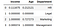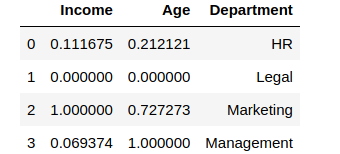You can see how the values were scaled. The minimum value among the columns became 0, and the maximum value was changed to 1, with other values in between. However, suppose we don’t want the income or age to have values like 0. Let us take the range to be (5, 10)

``````from sklearn.preprocessing import MinMaxScaler
scaler = MinMaxScaler(feature_range=(5, 10))

df_scaled[col_names] = scaler.fit_transform(features.values)
df_scaled
``````

This is what the output looks like: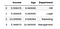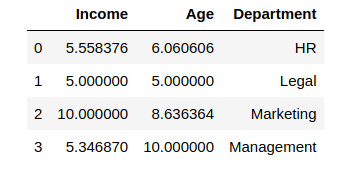Amazing, right? The min-max scaler lets you set the range in which you want the variables to be.

## Standard Scaler

Just like the MinMax Scaler, the Standard Scaler is another popular scaler that is very easy to understand and implement.

For each feature, the Standard Scaler scales the values such that the mean is 0 and the standard deviation is 1(or the variance).

x_scaled = x — mean/std_dev

However, Standard Scaler assumes that the distribution of the variable is normal. Thus, in case, the variables are not normally distributed, we

1. either choose a different scaler
2. or first, convert the variables to a normal distribution and then apply this scaler

Implementing the standard scaler is much similar to implementing a min-max scaler. Just like before, we will first import StandardScaler and then use it to transform our variable.

#feature-engineering #feature-scaling #scikit-learn #deep learning1597869360

## Feature Engineering with the help of Data Visualization

Why Feature Engineering is important?

The features in your data will directly influence the accuracy of your model. The better features gives good accuracy on your test data. The better the features that you choose, the better the results you will achieve.

## Feature Reduction In ML

The General view is adding more features increases the model/classifier’s performance.

Let us see why this is not the case:

• Curse of Dimensionlaity:

Choosing more number of features will usually degrade classifier’s performance.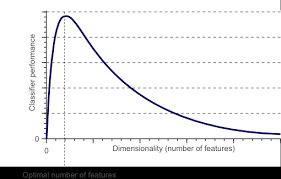CURSE OF DIMENSIONALITY

• Limited training data
• Limited Computational Resources
• addition of irrelevant/unnecessary features leads to increase in computational costs and decrease in performance.

## Feature Selection:

Having n initial features, it is possible to select (2^n ) combinations of features(2^n subsets are possible).

We just can’t go over all the possible (2^n) subsets.

Feature Selection is an optimization problem

• Search the space of possible subsets.
• Pick the one which best suits**.**

Evaluating the Feature Subset

#ai #data-science #deep-learning #feature-engineering #machine-learning #deep learning